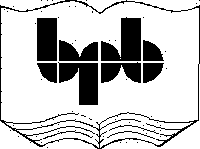5. The Blob Stream

b.il

.assembly b

{

}

.class  sequential public zzz

{

.method static vararg void pqr(int32 i, int64 j, int8 k )

{

.entrypoint

}

.method explicit void abc(int32 vij )

{

}

}

a.cs

using System;

using System.IO;

using System.Reflection;

using System.Configuration.Assemblies;

public class zzz

{

string [] paramnames;

public bool tablepresent(byte i)

{

int p = (int)(valid >> i) & 1;

byte [] sizes = {10,6,14,2,6,2,14,2,6,4,6,6,6,4,6,8,6,2,4,2,6,4,2,6,6,6,2,2,8,6,8,4,22,4,12,20,6,14,8,14,12,4};

for ( int j = 0 ; j < i ; j++)

{

int o = sizes[j] * rows[j];

tableoffset = tableoffset + o;

}

if ( p == 1)

return true;

else

return false;

}

public int ReadBlobIndex(byte [] a , int o)

{

int z = 0;

if ( offsetblob == 2)

z = BitConverter.ToUInt16 (a , o );

if ( offsetblob == 4)

z = (int)BitConverter.ToUInt32 (a , o );

return z;

}

public int ReadStringIndex(byte [] a , int o)

{

int z = 0;

if ( offsetstring == 2)

z = BitConverter.ToUInt16 (a , o );

if ( offsetstring == 4)

z = (int)BitConverter.ToUInt32 (a , o );

return z;

}

public string GetString(int starting)

{

int i = starting;

while (strings[i] != 0 )

{

i++;

}

System.Text.Encoding e = System.Text.Encoding.UTF8;

string  s = e.GetString(strings, starting , i - starting  );

return s;

}

public static void Main ()

{

zzz a = new zzz();

a.abc();

}

string [] tablenames;

int tableoffset ;

int [] rows;

int [] offset;

int [] ssize ;

byte [] strings;

byte [] us;

byte [] guid;

byte [] blob;

long valid ;

byte [][] names;

string [] streamnames;

int baseofcode;

int baseofdata ;

int sectiona;

int filea ;

byte heapsizes;

int offsetstring = 2;

int offsetblob = 2;

int offsetguid = 2;

public string GetStreamNames(byte [] b)

{

int i = 0;

while (b[i] != 0 )

{

i++;

}

System.Text.Encoding e = System.Text.Encoding.UTF8;

string  s = e.GetString(b , 0 , i  );

return s;

}

public void abc()

{

FileStream s = new FileStream("C:\\mdata\\b.exe",FileMode.Open);

s.Seek(60, SeekOrigin.Begin);

s.Seek(peoffset + 24 + 20 , SeekOrigin.Begin);

s.Seek(4, SeekOrigin.Current);

s.Seek(360, SeekOrigin.Begin);

int rva,size;

int where = rva%baseofcode + filea ;

s.Seek(where + 4 + 4, SeekOrigin.Begin);

where = rva%baseofcode + filea ;

s.Seek(where, SeekOrigin.Begin);

s.Seek(4 + 2 + 2 + 4 + 4 + 12 + 2, SeekOrigin.Current);

streamnames = new string;

offset = new int;

ssize  = new int;

names = new byte[];

names = new byte;

names = new byte;

names = new byte;

names = new byte;

names = new byte;

int i = 0; int j ;

for ( i = 0 ; i < streams ; i++)

{

j = 0;

byte bb ;

while ( true )

{

if ( bb == 0)

break;

names[i][j] = bb;

j++;

}

names[i][j] = bb;

streamnames[i] = GetStreamNames(names[i]);

while ( true )

{

if ( s.Position % 4 == 0 )

break;

if ( b != 0)

{

s.Seek(-1, SeekOrigin.Current);

break;

}

}

}

for ( i = 0 ; i < streams ; i++)

{

if ( streamnames[i]  == "#~" )

{

for ( int k = 0 ; k < ssize[i] ; k ++)

}

if ( streamnames[i]  == "#Strings" )

{

strings = new byte[ssize[i]];

for ( int k = 0 ; k < ssize[i] ; k ++)

}

if ( streamnames[i]  == "#US" )

{

us = new byte[ssize[i]];

for ( int k = 0 ; k < ssize[i] ; k ++)

}

if ( streamnames[i]  == "#GUID" )

{

guid = new byte[ssize[i]];

for ( int k = 0 ; k < ssize[i] ; k ++)

}

if ( streamnames[i]  == "#Blob" )

{

blob = new byte[ssize[i]];

for ( int k = 0 ; k < ssize[i] ; k ++)

}

}

if ( (heapsizes & 0x01) == 0x01)

{

offsetstring = 4;

}

if ( (heapsizes & 0x02) == 0x02 )

{

offsetguid = 4;

}

if ( (heapsizes & 0x08) == 0x08 )

{

offsetblob = 4;

}

tableoffset = 24;

rows = new int;

Array.Clear (rows, 0, rows.Length);

for ( int k = 0 ; k <= 63 ; k++)

{

int tablepresent = (int)(valid >> k ) & 1;

if ( tablepresent == 1)

{

tableoffset += 4;

}

}

tablenames = new String[]{"Module" , "TypeRef" ,            "TypeDef" ,"FieldPtr","Field", "MethodPtr","Method","ParamPtr" , "Param", "InterfaceImpl", "MemberRef", "Constant", "CustomAttribute", "FieldMarshal", "DeclSecurity", "ClassLayout", "FieldLayout", "StandAloneSig" , "EventMap","EventPtr", "Event", "PropertyMap", "PropertyPtr", "Properties","MethodSemantics",

"MethodImpl","ModuleRef","TypeSpec","ImplMap","FieldRVA",

"ENCLog","ENCMap","Assembly","AssemblyProcessor","AssemblyOS",

"AssemblyRef","AssemblyRefProcessor","AssemblyRefOS","File",

"ExportedType","ManifestResource","NestedClass",

"TypeTyPar","MethodTyPar"};

FillParamsArray();

DisplayMethodTable();

}

public void FillParamsArray()

{

int old = tableoffset;

bool b = tablepresent(8);

int offs = tableoffset;

tableoffset = old;

if ( b )

{

paramnames = new string[rows + 1];

for ( int k = 1 ; k <= rows ; k++)

{

short pattr = BitConverter.ToInt16 (metadata, offs);

offs += 2;

int sequence = BitConverter.ToInt16 (metadata, offs);

offs += 2;

offs += offsetstring;

string s = GetString(name);

paramnames[k] = s;

}

}

}

public void DisplayMethodTable()

{

int old = tableoffset;

bool b = tablepresent(6);

int offs = tableoffset;

tableoffset = old;

if ( b )

{

Console.WriteLine();

Console.WriteLine(tablenames);

for ( int k = 1 ; k <= rows ; k++)

{

int rva = BitConverter.ToInt32 (metadata, offs);

offs += 4;

MethodImplAttributes impflags = (MethodImplAttributes )BitConverter.ToInt16 (metadata, offs);

offs += 2;

int flags = (int)BitConverter.ToInt16 (metadata, offs);

offs += 2;

offs += offsetstring;

offs += offsetblob;

int param = BitConverter.ToInt16 (metadata, offs);

offs += 2;

Console.WriteLine("Name     : {0}", GetString(name));

string s = DisplayMethodSignature(signature , param , GetString(name) );

Console.WriteLine("Signature: #Blob[{0}] {1}",signature , s);

}

}

}

public string DisplayMethodSignature (int index , int param , string name)

{

string  s = "";

int count = blob[index];

s = s + count.ToString() + " ";

for ( int l = 1; l <= count ; l++)

s = s + blob[index+l].ToString() + " ";

byte [] blob1 = new byte[count];

Array.Copy(blob , index + 1 , blob1 , 0 , count);

byte firstfourbits = (byte)(blob1 & 0x0f);

if ( firstfourbits == 0x00)

s = s + " DEFAULT ";

if ( firstfourbits == 0x05)

s = s + " VARARG ";

if ( (blob1 & 0x20) == 0x20)

s = s + " HASTHIS ";

if ( (blob1 & 0x40) == 0x40)

s = s + " EXPLICIT ";

int paramcount = blob1;

s = s + "Count " + paramcount.ToString() + " " ;

s = s + " " + GetType(blob1) + " " ;

s = s + name + "(";

for ( int k = 1 ; k <= paramcount ; k++)

{

s = s + " " + GetType(blob1[2 + k]) + " " + paramnames[k] ;

if ( k != paramcount )

s = s + ",";

}

s = s + ")";

return s;

}

public string GetType(int b)

{

if ( b == 0x01)

return "void";

if ( b == 0x02)

return "boolean";

if ( b == 0x03)

return "char";

if ( b == 0x04)

return "byte";

if ( b == 0x05)

return "ubyte";

if ( b == 0x06)

return "short";

if ( b == 0x07)

return "ushort";

if ( b == 0x08)

return "int";

if ( b == 0x09)

return "uint";

if ( b == 0x0a)

return "long";

if ( b == 0x0b)

return "ulong";

if ( b == 0x0c)

return "float";

if ( b == 0x0d)

return "double";

if ( b == 0x0e)

return "string";

return "unknown";

}

}

Output

Method

Name     : pqr

Signature: #Blob 6 5 3 1 8 10 4  VARARG Count 3  void pqr( int i, long j, byte k)

Name     : abc

Signature: #Blob 4 96 1 1 8  DEFAULT  HASTHIS  EXPLICIT Count 1  void abc( int i)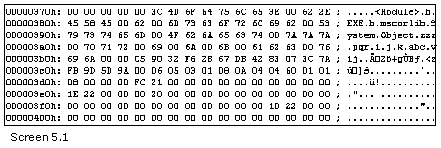The above example is the inaugural stride in deciphering the method signatures. This program is directed towards serving as a prototype for the entire chapter. The freshly introduced functions shall be appended at the end of the abc function. Two functions named FillParamsArray and DisplayMethodSignature have been added to the program.

To enable the display of the function signatures, the presence of parameter names is ineluctable, as can be seen in the output. At this stage, the function FillParamsArray method merely populates a string array with the names of each parameter. The code given earlier to read the Param table, is re-used to fill up an instance array called paramnames.

The eighth member in the row array determines the size of the array. In contrast to the array, which starts at 0, the row numbers always start at 1. Thus, the array size is increased by 1, so that the array offset is in sync with the row numbers.

Finally, at the culmination of the function, there is an array called paramnames, which contains the names of the parameters that are to be displayed as part of the function signature. The very essence of the above program is not the function DisplayMethodTable, but the function DisplayMethodSignature.

In the DisplayMethodTable function, we start out by reading the entire method table. However, only the name and the signature field that stores the offset of the method signature in the Blob stream are utilized.

Thereafter, the function DisplayMethodSignature is called with three parameters: an offset into the Blob heap, an offset into the param table (where the first parameter of this function is located), and finally, the name of the function as a string. But, the param offset would be replete with meaningful significance, only if the method signature identifies the parameters.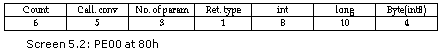As always, the first byte is the count byte, which has a value of six for the first function pqr. The count byte is stored in a variable called count. Then, an array called blob1 is created and the entire method signature, which is limited in size to the value of count, is copied into this byte array.

This approach simplifies and abridges the process of interpreting the method signature efficiently, since the array blob1 is used exclusively to store the signature, unlike the Blob that accommodates an assortment of other things too.

Following the count byte is a byte that contains two diverse types of information:

One relates to the calling convention.

The other talks about the 'this' pointer.

The variable named firstfourbits retrieves the first four bits of the first byte, which establishes the calling convention.

Of the four, if the first three bits have values of 0, the calling convention is the default one. If the value is 0x05, the calling convention is that of vararg. The vararg calling convention facilitates the passing of a variable number of arguments.

The second set of four bits identifies whether the 'this' pointer has been passed or not, and also whether the modifier 'explicit' has been used with the function or not. The 'this' pointer is never specified with static functions. As a result of this, the sixth bit is OFF, as seen in the pqr function, which is marked as static.

On the other hand, the function abc has the sixth bit ON, and it also employs the 'explicit' modifier.

The first byte of the method signature deals with calling conventions. The next byte provides a count of the number of parameters that the method uses. This value is stored in a variable called paramcount, and it is used to display all the parameters.

The third byte accommodates the return type, which has been assumed to be a simple one. The GetType function returns the type in a string format. Here, the parameters too are assumed to be holding simple value types.

The 'for' loop repeats the process, contingent on the number of parameters, thereby displaying all the parameters that the function takes. The GetType function is used to return the type and the paramarray array is used to provide the name of the array. This is the mechanism by means of which, the signature is displayed in a readable form.

We have made far too many assumptions about the method signature. Now that the basics have been well construed, let us build upon the above foundation and write a method that decodes the most complex of method signatures.

b.il

.assembly b

{

}

.class  sequential public zzz

{

.method static vararg class zzz pqr(int64 b , [out] int32& aa ,  class zzz c , class yyy d , int8 [6,7,3...9 , , ] d)

{

.entrypoint

}

}

.class yyy

{

}

The functions of FillParamsArray, DisplayMethodTable and DisplayMethodSignature have been modified. Further, the code specified below, should be placed after the GetType function, but before the closing brace of class zzz.

a.cs

string [] typedefnames;

string [] typerefnames;

public void FillParamsArray()

{

int old = tableoffset;

bool b = tablepresent(8);

int offs = tableoffset;

tableoffset = old;

if ( b )

{

paramnames = new string[rows + 1];

for ( int k = 1 ; k <= rows ; k++)

{

short pattr = BitConverter.ToInt16 (metadata, offs);

offs += 2;

int sequence = BitConverter.ToInt16 (metadata, offs);

offs += 2;

offs += offsetstring;

string s = GetString(name);

paramnames[k] = s;

}

}

old = tableoffset;

b = tablepresent(1);

offs = tableoffset;

tableoffset = old;

if ( b )

{

typerefnames = new string[rows+1];

for ( int k = 1 ; k <= rows ; k++)

{

short resolutionscope = BitConverter.ToInt16 (metadata , offs);

offs = offs + 2;

offs = offs + offsetstring;

offs = offs + offsetstring;

string s = GetString(name);

string s1 = GetString(nspace);

if ( s1.Length != 0)

s1 = s1 + ".";

s1 = s1 + s;

typerefnames[k] = s1;

}

}

old = tableoffset;

b = tablepresent(2);

offs = tableoffset;

tableoffset = old;

if ( b )

{

typedefnames = new string[rows+1];

for ( int k = 1 ; k <= rows ; k++)

{

TypeAttributes flags = (TypeAttributes)BitConverter.ToInt32 (metadata, offs);

offs += 4;

offs += offsetstring;

offs += offsetstring;

short cindex = BitConverter.ToInt16 (metadata, offs);

offs += 2;

int findex = BitConverter.ToInt16 (metadata, offs);

offs += 2;

int mindex = BitConverter.ToInt16 (metadata, offs);

offs += 2;

string s = GetString(name);

string s1 = GetString(nspace);

if ( s1.Length != 0)

s1 = s1 + ".";

s1 = s1 + s;

typedefnames[k] = s;

}

}

}

public void DisplayMethodTable()

{

int old = tableoffset;

bool b = tablepresent(6);

int offs = tableoffset;

tableoffset = old;

if ( b )

{

for ( int k = 1 ; k <= rows ; k++)

{

int rva = BitConverter.ToInt32 (metadata, offs);

offs += 4;

MethodImplAttributes impflags = (MethodImplAttributes )BitConverter.ToInt16 (metadata, offs);

offs += 2;

int flags = (int)BitConverter.ToInt16 (metadata, offs);

offs += 2;

offs += offsetstring;

offs += offsetblob;

int param = BitConverter.ToInt16 (metadata, offs);

offs += 2;

Console.WriteLine("Name     : {0}", GetString(name));

string s = DisplayMethodSignature(signature , param , GetString(name) );

Console.WriteLine("Signature: #Blob[{0}] {1}",signature , s);

}

}

}

public string DisplayMethodSignature(int index , int param , string name) {

string  s = "Count=";

int cb; int uncompressedbyte;

int count;

cb = CorSigUncompressData(blob , index , out uncompressedbyte);

Console.WriteLine("Count Byte cb={0} uncompresedbyte={1}", cb,uncompressedbyte);

count = uncompressedbyte;

s = s + count.ToString() + " Bytes ";

for ( int l = 0; l < count ; l++)

s = s + blob[index+l+cb].ToString() + " ";

byte [] blob1 = new byte[count];

Array.Copy(blob , index + cb  , blob1 , 0 , count);

index = 0;

cb = CorSigUncompressData(blob1 , index  , out uncompressedbyte);

Console.WriteLine("Calling Convention Byte cb={0} uncompresedbyte={1}", cb,uncompressedbyte);

s = s + GetCallingConvention(uncompressedbyte);

int paramcount;

index = index + cb;

cb = CorSigUncompressData(blob1 , index  , out uncompressedbyte);

Console.WriteLine("Parameter Count cb={0} uncompresedbyte={1}", cb,uncompressedbyte);

paramcount = uncompressedbyte;

s = s + "Number of Parameters " + paramcount.ToString() + "\n";

index = index + cb;

cb = CorSigUncompressData(blob1 , index  , out uncompressedbyte);

Console.WriteLine("Return Type cb={0} uncompresedbyte={1}", cb,uncompressedbyte);

string s1;

s1 = GetReturnType( blob1 , index  , out cb);

s = s + "Return Type:" + s1 + "\n";

index = index + cb;

s = s + "Signature " + name + "(";

for ( int l = 1 ; l <= paramcount ; l++)  {

cb = CorSigUncompressData(blob1 , index  , out uncompressedbyte);

int cb1 ;

int bytes = uncompressedbyte;

Console.WriteLine("Before GetElementType {0}" , bytes);

s1 = GetElementType(blob1 , bytes , index , out cb1);

index = index + cb + cb1;

s = s + s1 + " " + paramnames[l] + " ";

if ( l != paramcount)

s = s + "," ;

}

s = s + ")\n";

return s;

}

public string GetCallingConvention ( int uncompressedbyte )

{

int firstbyte = uncompressedbyte;

byte firstfourbits = (byte)(firstbyte & 0x0f);

string s = "\nCalling Convention ";

if ( firstfourbits == 0x00)

s = s + " DEFAULT ";

if ( firstfourbits == 0x05)

s = s + " VARARG ";

if ( (firstbyte & 0x20) == 0x20)

s = s + " HASTHIS ";

if ( (firstbyte & 0x40) == 0x40)

s = s + " EXPLICIT ";

s = s + "\n";

return s;

}

public string GetElementType(byte [] b , int bytes , int index , out int cb1) {

cb1 = 0;

string s = "";

if ( bytes <= 0x0e )

{

cb1 = 0;

s = GetType(bytes);

}

if ( bytes == 0x12 )

{

s = GetClassType(b , bytes , index , out cb1);

}

if ( bytes == 0x14)

{

s = GetArrayType(b , bytes , index , out cb1);

}

if ( bytes == 0x10)

{

s = GetReferenceType(b , bytes , index , out cb1);

}

return s;

}

public string GetReferenceType( byte [] b , int bytes , int index , out int cb1)

{

string s = "[Out] ";

int total = 1;

int uncompressedbyte;

int cb = CorSigUncompressData(b , index + 1 , out uncompressedbyte);

int cb2;

s = s + GetElementType(b , uncompressedbyte , index + 1 , out cb2);

total = total + cb2;

cb1 = total;

return s;

}

public string GetArrayType(byte [] b , int bytes , int index , out int cb1) {

string s ;

int total = 1;

int uncompressedbyte;

int rank;

int numsizes;

int cb = CorSigUncompressData(b , index + 1 , out uncompressedbyte);

total = total + cb;

s = GetElementType(b,uncompressedbyte , index +1 , out cb1);

total = total + cb1;

s = s + " [";

cb = CorSigUncompressData(b , index + total, out uncompressedbyte);

total = total + cb;

rank = uncompressedbyte;

Console.WriteLine("Rank {0}" , rank);

cb = CorSigUncompressData(b , index + total, out uncompressedbyte);

total = total + cb;

numsizes = uncompressedbyte;

Console.WriteLine("Num Sizes {0}" , numsizes );

int [] sizearray = new int[numsizes];

for ( int l = 1 ; l <= numsizes ; l++)

{

cb = CorSigUncompressData(b , index + total, out uncompressedbyte);

total = total + cb;

sizearray[l-1] = uncompressedbyte;

}

cb = CorSigUncompressData(b , index + total, out uncompressedbyte);

total = total + cb;

int bounds = uncompressedbyte;

int [] boundsarray = new int[bounds];

for ( int l = 1 ; l <= bounds ; l++)

{

cb = CorSigUncompressData(b , index + total, out uncompressedbyte);

total = total + cb;

int ulSigned = uncompressedbyte & 0x1;

uncompressedbyte  = uncompressedbyte >> 1;

Console.WriteLine(ulSigned);

if (ulSigned == 1)

{

if (cb == 1)

{

uncompressedbyte =  (int) (uncompressedbyte | 0xffffffc0);

}

else if (cb == 2)

{

uncompressedbyte = (int) (uncompressedbyte | 0xffffe000) ;

}

else

{

uncompressedbyte = (int)(uncompressedbyte | 0xf0000000);

}

}

boundsarray[l-1] = uncompressedbyte ;

}

Console.Write("Size Array ");

for ( int l = 1 ; l <= numsizes; l++)

{

Console.Write("{0} ", sizearray[l-1]);

}

Console.WriteLine();

Console.Write("Bounds Array ");

for ( int l = 1 ; l <= bounds ; l++)

{

Console.Write("{0} ", boundsarray[l-1]);

}

Console.WriteLine();

for ( int l = 0 ; l < bounds ; l++)

{

int upper = boundsarray[l] + sizearray[l] - 1 ;

if ( boundsarray[l] == 0 && sizearray[l] != 0 )

{

s = s + sizearray[l] ;

if ( l != bounds -1 )

s = s + ",";

}

if (boundsarray[l] == 0 && sizearray[l] == 0)

s = s + "," ;

if (boundsarray[l] != 0 && sizearray[l] != 0)

s = s + boundsarray[l] + "..." + upper.ToString() + "," ;

}

int leftover = rank - numsizes;

for ( int l = 1 ; l < leftover ; l++)

s = s + ",";

s = s + "]";

cb1 = total-1;

return s;

}

public string GetClassType(byte [] b , int bytes , int index , out int cb1) {

int uncompressedbyte;

int cb = CorSigUncompressData(b , index + 1 , out uncompressedbyte);

Console.WriteLine("Token Count cb={0} uncompresedbyte={1}", cb,uncompressedbyte);

byte table = (byte)(uncompressedbyte & 0x03);

int ind = uncompressedbyte >> 2;

Console.WriteLine("Token Table={0} index={1}", table ,ind);

string s1= "";

if ( table == 1)

s1 = typerefnames[ind];

if ( table == 0)

s1 = typedefnames[ind];

cb1 = cb;

return s1;

}

public string GetReturnType(byte [] b , int index , out int cb)

{

string s = "";

cb = 0;

if ( b[index] <= 0x0e )

{

s = GetType(b[index]);

cb = 1;

}

if ( b[index] == 0x12 )

{

int cb1;

int uncompressedbyte ;

cb1 = CorSigUncompressData( b , index +1 , out uncompressedbyte );

byte table = (byte)(uncompressedbyte & 0x03);

int ind = uncompressedbyte >> 2;

if ( table == 1)

s = typerefnames[ind];

if ( table == 0)

s = typedefnames[ind];

cb = cb1 + 1;

}

return s;

}

public int CorSigUncompressData( byte [] b , int index , out int answer) {

int cb = 0;

if ( (b[index] & 0x80) == 0x00)

{

cb = 1;

}

if ( (b[index] & 0xC0) == 0x80)

{

cb = 2;

answer = ((b[index] & 0x3f) <<8 ) |  b[index+1];

}

if ( (b[index] & 0xE0) == 0xC0)

{

cb = 2;

answer = ((b[index] & 0x1f) <<24 ) |  (b[index+1] << 16) |  (b[index+2] << 8) | b[index+3];

}

return cb;

}

Output

Name     : pqr

Count Byte cb=1 uncompresedbyte=22

Calling Convention Byte cb=1 uncompresedbyte=5

Parameter Count cb=1 uncompresedbyte=5

Return Type cb=1 uncompresedbyte=18

Before GetElementType 10

Before GetElementType 16

Before GetElementType 18

Token Count cb=1 uncompresedbyte=8

Token Table=0 index=2

Before GetElementType 18

Token Count cb=1 uncompresedbyte=9

Token Table=1 index=2

Before GetElementType 20

Rank 5

Num Sizes 3

0

0

0

Size Array 6 7 7

Bounds Array 0 0 3

Signature: #Blob Count=22 Bytes 5 5 18 8 10 16 8 18 8 18 9 20 4 5 3 6 7 7 3 0 0 6

Calling Convention  VARARG

Number of Parameters 5

Return Type:zzz

Signature pqr(long b ,[Out] int aa ,zzz c ,yyy d ,byte [6,7,3...9,,] d )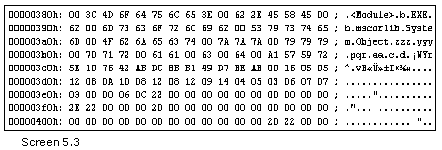Let us commence with the IL file first. We have attempted to imbue it with as much complexity as possible. The pqr function takes five parameters: the first one 'b' is a simple type; the second, i.e. 'aa' is an out parameter; the third one is the zzz type, the fourth is the yyy type, and finally, the last parameter 'd' is an array type. The return value is a zzz object. However, this is not the last word on the method, since it can be made considerably more complex.

In the FillParamsArray method, two more string arrays called typerefnames and typedefnames have been created, to store the names of the types present in the TypeRef table and TypeDef table, respectively. The types that we create or refer to in the method signature, are stored in these tables. These two arrays are employed in a manner akin to the paramarray.

Apart from the 3 functions of FillParamsArray, DisplayMethodTable and DisplayMethodSignature, the code is left unaltered from the earlier program. We have also eliminated the superfluous code that displays the attributes, etc.

The DisplayMethodSignature method is passed the starting offset of the method signature in the Blob stream. This is the most vital parameter, since without the signature index, there is no pathway to forge ahead. Along with the signature, the names of the parameters also need to be displayed. Hence, we have the starting param table index as the second parameter.  Finally, the name of the function is displayed through the third and last parameter.

The primary objective of this function is to return a string that reveals the method signature.

To achieve this, we have introduced multiple WriteLine statements. We have split the code into several methods, as far as possible, so as to make them worthy of reuse. However, on the flipside of it, while making headway from one method to the next, there is a likelihood of your getting utterly baffled and going adrift. Nonetheless, on the brighter side of it, it renders it easy to understand the code, without the inevitable need for repetition.

Before we move on to unveil the newly introduced code in the program, a few things summon our immediate attention.

The first byte of any entity in the Blob stream is a count byte. For a number of reasons, we have always gone by the assumption that the count is a byte; and upto this point, our conviction has not been disproved. However, the only apparent shortcoming of a byte is that, it is restricted in its capacity, i.e. it can only accommodate a number ranging from 0 to 255, which is a total of 256 or 2^8 numbers.

The quandary here is that if the signature size extends beyond 255 bytes, it is incapable of being represented in a single byte. It requires two bytes to accommodate numbers ranging between 0 to 65535, which is a total of 65536 or 2 ^16 numbers. At certain times, even this range falls woefully short. In such cases, an int is pressed into action, which has a capacity of storing upto 2 ^ 32 numbers.

However, on most occasions, the number lies within the range of 0 to 127, and only on certain occasions, does it extend upto the 1000s. Therefore, it is an exercise in futility to reserve an int in order to store the number, since it would entail dissipation of considerable space. Thus, the designers of the metadata decided to compress each byte before storing it in the metadata streams.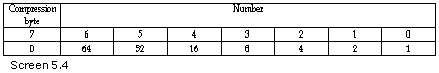This leads to a smaller stream size. The speed penalty that arises as a result of the additional time spent in compressing and decompressing the bytes is insignificant.

The following approach is applied:The first bit of the first byte is checked. If it is 0, it signifies that the next 7 bits represent the number. Thus, for numbers between 0 and 127 or 0x00 to 0x7f, a single byte is used.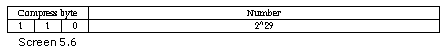Since the count byte generally falls within this range, there would be tremendous savings on the space occupied. For larger numbers, the first bit is switched ON. In such cases, the subsequent bit is checked. If this bit is zero, it signifies that the next 14 bits store the number. Thus, numbers from 128 to 16383 (2^14-1) or from 0x80 to 0x3fff can be accommodated in 14 bits.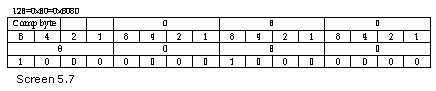Finally, if the first bit is set to 1 and the second bit is also set to 1, the third bit is checked. If the third bit is 0, it implies that the count member can store numbers upto 0x1FFFFFFF or 536870911, using 29 bits.

Let us minutely examine an example from the documentation. A number such as 0x03 or 0x7f is stored using 8 bits. A number like 128 or 0x80, which is larger than 127, will be stored using 16 bits, in the form of 0x8080.

This is because, the 16th bit is set, the 15th bit is clear, and the remaining 14 bits accommodate the value. In the same vein, 0x2E57 is stored as 0xAE57, and the largest number that can be accommodated in two bytes, i.e. 0x3FFF, is stored as BFFF. The number 0x4000 requires 4 bytes, since it is larger than 0x3FFF. It is stored as C000 4000, with the 31st and 30th bit set, and the 29th bit clear.

Finally, the largest number that can be represented in compressed streams is 0x1FFF FFFF. It is stored as 0xDFFFFFFF. The other glitch is that we are examining the top most bits, while in the little endian system, the low byte is stored first. This becomes a hindrance, since our intention is to read the high bits of the high byte.

Hence, all the values are stored in the reverse order, i.e. in big endian system, where the high byte is stored first. For instance, the number 515 is stored as 3 followed by 2 in the little endian format, and as 2 followed by a 3 in the big endian format. Every time a value is read, a check is to be performed to ascertain whether the byte is compressed or not. If it is compressed, it needs to be uncompressed.

The value stored in these bytes is an int, which represents the size or the number of bytes that are to be picked up from the Blob area. Thus, we need a function that would accept an array of bytes, the starting position or the index of the byte, and an int to store the uncompressed byte. In addition, we would also wish to store the return value and the number of bytes that are finally used.

Writing a function that caters to all these specifications was indeed a Herculean task. So, we scrutinized a program called MetaInfo, which is provided with the FrameWorkSDK in the Tool Developers code. This example also displays the information present in the Metadata tables. It is written in managed C++. The only handicap that comes as a package deal with this program is that, it does not bestow us with raw access to the bytes in the table.

However, it furnishes ample information on aspects such as the method signature, etc. In the MetaInfo program, the method CorSigUncompressData is called for uncompressing the bytes. We also decided to call our method by the same name. This method takes three parameters, i.e. the Blob array, an offset into the array where the signature begins, and finally, an out parameter that stores the final uncompressed value. The return value divulges whether the un-compression was using 1 or 2 or 4 bytes.

Let us now understand the workings of the method CorSigUncompressData.

The index parameter of the function helps in inspecting the byte in the Blob stream. The first bit is examined to see if it is 0 or not, by ANDing it with 0x80. If the answer is 0, it denotes that the last bit is 0, and not 1. This signifies that the value falls within the range of 0 to 127, and that it is stored in a single byte. We set the out parameter answer to the value contained in the byte array. Then, we set the return value to 1, signifying that a single byte has been consumed upto this point. The going has been great so far!

By ANDing the byte in the array with 0xc0, it can be ascertained whether the last two bits are ON or not. If the result is 0x80, it indicates that the last bit was ON, i.e. the last bit is set, and the second last bit is clear. This merely signifies that the next two bytes store the uncompressed byte, and thus, the cb variable is set to 2.

Then, in the high byte, the 15th and the 14th bit are set to 0 by bitwise ANDing them with 3f. The resulting 6 bits are left-shifted by 8 bits, so that they occupy bytes 8 to 15. Then, they are ORed with the next byte in the byte array, so that the first 8 bits get filled up.

If the value extends beyond the valid limit, a final check is made to establish if the last two bits are set or not, and also whether the third last bit is clear or not. Thus, the byte is ANDed with 0xe0, which is the bit pattern for the last 3 bits being ON. If the answer is 0xc0, i.e. if the last two bits are ON and the third bit is OFF, it indicates that the size of the signature is limited to 4 bytes.

Since the default is set to a big endian machine, the last three bits are masked OFF, and the 24 bits are right-shifted to occupy the high byte of the long. Thereafter, we access the next byte of the byte array and right-shift it by 16, so that it occupies the second last byte. The third byte is only shifted by 8, while the fourth byte is not shifted at all. They are ORed together to obtain the complete byte.

The cb variable is then set to 4, since 4 bytes are consumed. This compression method provides 29 usable bits.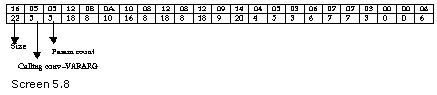Thus, each time the decompression method is called, the variable index identifies the offset into the Blob array. The return value of this function is stored in a variable called cb in DisplayMethodSignature.

Thereafter, the value of the cb variable is added to the index variable, since there is no other means of determining the number of bytes that were uncompressed from the Blob stream. The count variable is initialized to the value stored in the variable named uncompressedbyte. In our case, the signature is 22 bytes long. Then, the next 22 bytes are displayed merely to reveal the method signature in its raw form.

We would advise you to pause here, and add about 120 parameters to the above function. This would increase the count value to more than 127, and then, you could then effortlessly verify the output. The uncompress method conjures up its magic by setting the value of cb to 2.

Like before, the Array.Copy function provides a clean copy of the signature bytes in the blob1 array, and the index variable is set to 0, since that is where the first byte begins.

The byte following the count variable represents the calling convention. To identify the calling convention, the function GetCallingConvention is used, which decodes the first byte and returns a string value. The myriad complexities involved in the calling convention shall be delved upon a little later.

The third entity is the param count. It shows a value of 5, indicating the presence of five parameters. This value is stored in the variable named paramcount. Each time a byte is read, it is first uncompressed and then used.

The next byte is the return type. To decode the return type, the function GetReturnType is called. This function performs a specific job, and then, returns a string value.  The last parameter to this function divulges the number of bytes consumed by the return type. Besides, just like before, the function is passed the Blob array and the index position at which the return byte begins.

The function GetReturnType first essays to ascertain if the type is a simple type, i.e. whether the value is less than or equal to 0x0e, or not. If it is so, then the function GetType is exploited to decode this simple type and to set the cb variable to 1. However, presently, the return byte number is 18. This number represents a class; and thus, it ratifies the fact that the type is a class.

The byte following the return type furnishes the details of the class, which is stored as a token. These details of the class could emanate from any one of the three tables named TypeRef, TypeDef and TypeSpec.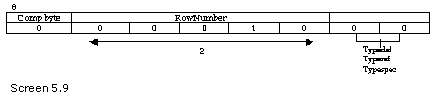Compression is the maxim of the metadata world. Therefore, the token, which represents the table name and index, is built in a very compact manner. The first two bits of the token select the table that is to be indexed into, while the remaining five bits allocate the row numbers in the table. The number of residual bits is five, and not six, because the first bit checks whether the row number takes up one byte or two bytes.

An index into three tables, upto the row number of 32, can be expressed as a byte. But, if the row number stretches beyond 32, then two bytes are used to store the token. Thus, firstly the row and table are compressed, and then, this token is compressed further. In our case, the token value is 8 and the first two bits are marked as 0. Thus, it indexes into the TypeDef table.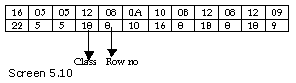For the next parameter, the index value is attained by right-shifting the token by 2 bits. Resultantly, the index is the second row of the type ref table, which is the class zzz.

The reverse coding is as follows:

We first take a row index and left-shift it by 2. Then, the two bits of the table are ORed, and finally, the value is compressed. The cb value is obviously cb1 + 1. It can either be 1, 2 or 4. The code for deciphering a class type can be placed in a separate method, to facilitate its reuse.

Now, we arrive at the nucleus of the program.

Following the return type are the parameters. A 'for' loop is used to iterate across all the parameters. Moreover, all parameters are stored back to back, with no count byte in the middle. The cb1 variable stores the number of bytes that the parameter needs.

In the loop, the byte is first uncompressed, and then, it is passed on to the GetElementType function, which decodes it. The function returns the parameter in the form of a sting.

The GetElementType method takes three items:

A byte array, which is the actual uncompressed byte.

An index called 'index' where the previous byte had resided.

The out parameter.

The job of this function is to call other functions, contingent upon the actual value of the byte called 'bytes'. As before, if the value is less than 0x0e, the GetType method is called. If it is 0x12, the function GetClassType is called, which executes the actual decoding.

The code specified in this function is similar to that contained in the GetReturnType function. We have reiterated it here in order to simplify it, resulting in enhanced comprehension.

The index variable points to the byte 0x0a, which represents a 'long'. This is the type for the first parameter. The next byte is 16. If the type displayed is 16, it indicates that it is a reference type, and has a metadata token following it. A reference type comes into play when the words 'ref' or 'out' are used in C#, or when the word [out] is used in IL. After displaying the word 'out', the next byte, which is the metadata token, is examined to unearth the table and row index.

Following the ref parameter are the two parameters of type zzz and yyy. Thus, the GetElementType function takes a byte and identifies the type. It could either be a simple type or a complicated one. If the value is 20, then the type is an array.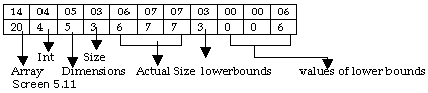For an array, the function GetArrayType is implemented with the same set of four parameters.

The first byte of the array signature is the data type of the array. As usual, the GetElementType function is applied to discern the type. The value of 4 indicates that the type is an int. Then, the next byte is the rank of the array. The rank represents the number of dimensions that an array owns. In this case, the array is in possession of five dimensions.

The task of the next byte of numsizes is to fathom how many dimensions have a size. In our case, only 3 out of 5 dimensions have sizes. The last two dimensions have no size whatsoever. This is perfectly legal.

Since the next couple of bytes contain the actual sizes of each of the dimensions, an array of ints, which is equal to numsizes, is created. If the array has an index ranging from 6…8, the size is 3. It is so because both the lower as well as the upper dimensions are also included. In b.il, the third dimension has been specified as 3…9; thus, the size is shown as 7.

This field is also known as the size of the array. If no dimensions have been specified, the size happens to be zero. If it is a single integer with no upper and lower bounds, then the size byte is the dimension of the array. In this case, first the sizearray is filled up, and then, an array called boundsarray is created, which contains the lower bound.

The byte that follows the sizes is comprised of the number of lower bounds, which is 3 in our case. This number is identical to the size byte. These bytes are the lower bounds for the dimensions, whose size has been specified. If the value is displayed as zero, it signifies that no lower bound has been specified.

The third array is seen with a value of 6, but in the IL file, it is reflected as 3. This is because the numbers are signed; therefore, they need to be uncompressed first. Thus, the first bit is checked.  Since it is unset, the bits are simply right-shifted by 1, thereby dropping off the first bit.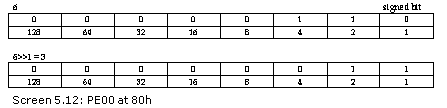If the first bit is set, then, depending upon the number of bytes that are compressed, certain bits are masked off.

The two arrays are displayed as sanity checks using the 'for' loop. They have the bounds variable as the main key.

Now, we need to place the dimensions of the array.

First, the upper bound is to be computed, which is calculated as the lower bound plus the size minus 1. The upper and lower bounds should then be placed in the return string. To accomplish this, first the bounds array is checked to ascertain if it is zero, and the size array is confirmed to be non-zero. If the condition results in true, it signifies the fact that the upper and lower bound have a simple size. The 'if' statement handles the last comma.

If both the arrays contain zero, it implies that the lower and upper bounds are present. Hence, they are to be displayed with three dots.

Finally, as a space saving feature, all the dimensions specified at the end are not placed in the two arrays. The difference between the rank and numsizes determines the number of empty commas needed in the array. Placing zeroes at the end of the two arrays is absurd and void of all sense.

Local Variables Signatures

b.cs

using System;

public class zzz

{

public static void Main()

{

}

public unsafe void abc()

{

int  j;

}

}

struct yyy

{

}

class xxx

{

public int x;

}

>csc b.cs /unsafe

a.cs

using System;

using System.IO;

using System.Reflection;

using System.Configuration.Assemblies;

public class zzz

{

string [] paramnames;

string [] typerefnames ;

string [] typedefnames ;

public bool tablepresent(byte i)

{

int p = (int)(valid >> i) & 1;

byte [] sizes = {10,6,14,2,6,2,14,2,6,4,6,6,6,4,6,8,6,2,4,2,6,4,2,6,6,6,2,2,8,6,8,4,22,4,12,20,6,14,8,14,12,4};

for ( int j = 0 ; j < i ; j++)

{

int o = sizes[j] * rows[j];

tableoffset = tableoffset + o;

}

if ( p == 1)

return true;

else

return false;

}

public int ReadBlobIndex(byte [] a , int o)

{

int z = 0;

if ( offsetblob == 2)

z = BitConverter.ToUInt16 (a , o );

if ( offsetblob == 4)

z = (int)BitConverter.ToUInt32 (a , o );

return z;

}

public string GetType(int b)

{

if ( b == 0x01)

return "void";

if ( b == 0x02)

return "boolean";

if ( b == 0x03)

return "char";

if ( b == 0x04)

return "byte";

if ( b == 0x05)

return "ubyte";

if ( b == 0x06)

return "short";

if ( b == 0x07)

return "ushort";

if ( b == 0x08)

return "int";

if ( b == 0x09)

return "uint";

if ( b == 0x0a)

return "long";

if ( b == 0x0b)

return "ulong";

if ( b == 0x0c)

return "float";

if ( b == 0x0d)

return "double";

if ( b == 0x0e)

return "string";

return "unknown";

}

public static void Main ()

{

zzz a = new zzz();

a.abc();

}

string [] tablenames;

int tableoffset ;

int [] rows;

int [] offset;

int [] ssize ;

byte [] strings;

byte [] us;

byte [] guid;

byte [] blob;

long valid ;

byte [][] names;

string [] streamnames;

int baseofcode;

int baseofdata ;

int sectiona;

int filea ;

byte heapsizes;

int offsetstring = 2;

int offsetblob = 2;

int offsetguid = 2;

public string GetStreamNames(byte [] b)

{

int i = 0;

while (b[i] != 0 )

{

i++;

}

System.Text.Encoding e = System.Text.Encoding.UTF8;

string  s = e.GetString(b , 0 , i  );

return s;

}

public void abc()

{

FileStream s = new FileStream("C:\\mdata\\b.exe",FileMode.Open);

s.Seek(60, SeekOrigin.Begin);

ii = ii + 4 + 16;

ii = ii + 24;

s.Seek(ii, SeekOrigin.Begin);

s.Seek(4, SeekOrigin.Current);

ii = 52;

s.Seek(ii, SeekOrigin.Current);

int rva,size;

ii = 14 *8 ;

s.Seek(ii, SeekOrigin.Current);

//Console.WriteLine("CLI Header RVA={0} Size={1}" , rva.ToString("X"),

size.ToString("X");

int where ;

if ( filea != sectiona)

where = rva%baseofcode + filea ;

else

where = rva;

s.Seek(where + 4 + 4, SeekOrigin.Begin);

if ( filea != sectiona)

where = rva%baseofcode + filea ;

else

where = rva;

s.Seek(where, SeekOrigin.Begin);

s.Seek(4 + 2 + 2 + 4 + 4 + 12 + 2, SeekOrigin.Current);

streamnames = new string;

offset = new int;

ssize  = new int;

names = new byte[];

names = new byte;

names = new byte;

names = new byte;

names = new byte;

names = new byte;

int i = 0; int j ;

for ( i = 0 ; i < streams ; i++)

{

j = 0;

byte bb ;

while ( true )

{

if ( bb == 0)

break;

names[i][j] = bb;

j++;

}

names[i][j] = bb;

streamnames[i] = GetStreamNames(names[i]);

while ( true )

{

if ( s.Position % 4 == 0 )

break;

if ( b != 0)

{

s.Seek(-1, SeekOrigin.Current);

break;

}

}

}

for ( i = 0 ; i < streams ; i++)

{

if ( streamnames[i]  == "#~" )

{

for ( int k = 0 ; k < ssize[i] ; k ++)

}

if ( streamnames[i]  == "#Strings" )

{

strings = new byte[ssize[i]];

for ( int k = 0 ; k < ssize[i] ; k ++)

}

if ( streamnames[i]  == "#US" )

{

us = new byte[ssize[i]];

for ( int k = 0 ; k < ssize[i] ; k ++)

}

if ( streamnames[i]  == "#GUID" )

{

guid = new byte[ssize[i]];

for ( int k = 0 ; k < ssize[i] ; k ++)

}

if ( streamnames[i]  == "#Blob" )

{

blob = new byte[ssize[i]];

for ( int k = 0 ; k < ssize[i] ; k ++)

}

}

if ( (heapsizes & 0x01) == 0x01)

{

offsetstring = 4;

}

if ( (heapsizes & 0x02) == 0x02 )

{

offsetguid = 4;

}

if ( (heapsizes & 0x08) == 0x08 )

{

offsetblob = 4;

}

tableoffset = 24;

rows = new int;

Array.Clear (rows, 0, rows.Length);

for ( int k = 0 ; k <= 63 ; k++)

{

int tablepresent = (int)(valid >> k ) & 1;

if ( tablepresent == 1)

{

tableoffset += 4;

}

}

FillParamsArray();

DisplayStandAloneSigTable();

}

public void DisplayStandAloneSigTable ()

{

int old = tableoffset;

bool b = tablepresent(17);

int offs = tableoffset;

tableoffset = old;

if ( b )

{

for ( int k = 1 ; k <= rows ; k++)

{

offs += offsetblob;

Console.WriteLine("Row {0}" , k);

byte count = blob[index];

string s = DisplayVariablesSignature (index);

Console.WriteLine(s);

}

}

}

public string DisplayVariablesSignature(int index)

{

string  s = "Count=";

int cb; int uncompressedbyte;

int count;

cb = CorSigUncompressData(blob , index , out uncompressedbyte);

count = uncompressedbyte;

s = s + count.ToString() + " Bytes ";

for ( int l = 1; l <= count ; l++)

s = s + blob[index+l+cb-1].ToString() + " ";

byte [] blob1 = new byte[count];

Array.Copy(blob , index + 1 + cb -1  , blob1 , 0 , count);

index = 0;

cb = CorSigUncompressData(blob1 , index  , out uncompressedbyte);

if ( uncompressedbyte != 0x07)

{

s = "Error";

return s;

}

index = index + cb;

int paramcount;

cb = CorSigUncompressData(blob1 , index  , out uncompressedbyte);

paramcount = uncompressedbyte;

index = index + cb;

for ( int l = 1 ; l <= paramcount ; l++)

{

cb = CorSigUncompressData(blob1 , index  , out uncompressedbyte);

int cb1 ;

int bytes = uncompressedbyte;

string s1 = GetElementType(blob1 , bytes , index , out cb1);

index = index + cb + cb1;

s = s + s1 ;

if ( l != paramcount )

s = s + " , ";

}

return s;

}

public string GetPinnedType( byte [] b , int bytes , int index , out int cb1)

{

string s = "";

int total = 1;

int uncompressedbyte;

int cb = CorSigUncompressData(b , index + 1 , out uncompressedbyte);

int cb2;

s = "Pinned " + GetElementType(b , uncompressedbyte , index + 1 , out cb2) ;

total = total + cb2;

cb1 = total;

return s;

}

public string GetPointerType( byte [] b , int bytes , int index , out int cb1)

{

string s = "";

int total = 1;

int uncompressedbyte;

int cb = CorSigUncompressData(b , index + 1 , out uncompressedbyte);

int cb2;

s = GetElementType(b , uncompressedbyte , index + 1 , out cb2) + " *" ;

total = total + cb2;

cb1 = total;

return s;

}

public string GetReferenceType( byte [] b , int bytes , int index , out int cb1)

{

string s = "[ByRef] ";

int total = 1;

int uncompressedbyte;

int cb = CorSigUncompressData(b , index + 1 , out uncompressedbyte);

int cb2;

s = s + GetElementType(b , uncompressedbyte , index + 1 , out cb2);

total = total + cb2;

cb1 = total;

return s;

}

public string GetClassType(byte [] b , int bytes , int index , out int cb1)

{

int uncompressedbyte;

int cb = CorSigUncompressData(b , index + 1 , out uncompressedbyte);

Console.WriteLine("Token Count cb={0} uncompresedbyte={1}", cb,uncompressedbyte);

byte table = (byte)(uncompressedbyte & 0x03);

int ind = uncompressedbyte >> 2;

Console.WriteLine("Token Table={0} index={1}", table ,ind);

string s1= "";

if ( table == 1)

s1 = typerefnames[ind];

if ( table == 0)

s1 = typedefnames[ind];

cb1 = cb;

return s1;

}

public string GetValueType(byte [] b , int bytes , int index , out int cb1)

{

int uncompressedbyte;

int cb = CorSigUncompressData(b , index + 1 , out uncompressedbyte);

byte table = (byte)(uncompressedbyte & 0x03);

int ind = uncompressedbyte >> 2;

string s1= "";

if ( table == 1)

s1 = typerefnames[ind];

if ( table == 0)

s1 = typedefnames[ind];

cb1 = cb;

return s1;

}

public string GetReturnType(byte [] b , int index , out int cb)

{

string s = "";

cb = 0;

if ( b[index] <= 0x0e )

{

s = GetType(b[index]);

cb = 1;

}

if ( b[index] == 0x12 )

{

int cb1;

int uncompressedbyte ;

cb1 = CorSigUncompressData( b , index +1 , out uncompressedbyte );

byte table = (byte)(uncompressedbyte & 0x03);

int ind = uncompressedbyte >> 2;

if ( table == 1)

s = typerefnames[ind];

if ( table == 0)

s = typedefnames[ind];

cb = cb1 + 1;

}

return s;

}

public int CorSigUncompressData( byte [] b , int index , out int answer)

{

int cb = 0;

if ( (b[index] & 0x80) == 0x00)

{

cb = 1;

}

if ( (b[index] & 0xC0) == 0x80)

{

cb = 2;

answer = ((b[index] & 0x3f) <<8 ) |  b[index+1];

}

if ( (b[index] & 0xE0) == 0xC0)

{

cb = 2;

answer = ((b[index] & 0x1f) <<24 ) |  (b[index+1] << 16) |  (b[index+2] << 8) | b[index+3];

}

return cb;

}

public string GetElementType(byte [] b , int bytes , int index , out int cb1)

{

cb1 = 0;

string s = "";

if ( bytes <= 0x0e )

{

cb1 = 0;

s = GetType(bytes);

}

if ( bytes == 0x12 )

{

s = GetClassType(b , bytes , index , out cb1);

}

if ( bytes == 0x14 || bytes == 0x1d)

{

//s = GetArrayType(b , bytes , index , out cb1);

}

if ( bytes == 0x10)

{

s = GetReferenceType(b , bytes , index , out cb1);

}

if ( bytes == 0x0f)

{

s = GetPointerType(b , bytes , index , out cb1);

}

if ( bytes == 0x11 )

{

s = GetValueType(b , bytes , index , out cb1);

}

if ( bytes == 0x45 )

{

s = GetPinnedType(b , bytes , index , out cb1);

}

if ( bytes == 0x1c )

{

s = "System.Object ";

}

if ( bytes == 0x16 )

{

s = "System.TypedReference ";

}

if ( bytes == 0x18 )

{

s = "System.IntPtr ";

}

if ( bytes == 0x19 )

{

s = "System.UIntPtr ";

}

return s;

}

Output

Row 1

Count=3 Bytes 7 1 8 int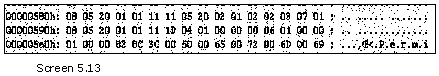The above example does two things:

Secondly, it explains signatures in a superior manner.

Each time a local variable is created in a function, a row gets added to the StandAloneSig table. Since the main function is devoid of local variables, no rows are added in the table. This table has a single field, which is the signature. No other metadata table references it, nor does it have to reference any other metadata table.

The b.cs file embodies an 'unsafe' function. Hence, it has to be compiled with the 'unsafe' option. Also, there is a variable i in the function. Before we go on to expound the signature any further, let us glimpse at the alterations bought about in the abc function.

In the earlier programs, the file pointer was positioned at the offset of 360, which had the Data Directory entry for the CLI header. This approach works extremely well for the IL Assembler and the C# compiler, since both these products have the PE header starting at the offset of 128.

Most compilers that we have worked with use a standard program, which runs whenever a program is executed under DOS. However, such is not the case with the managed C++ compiler. Thus, we cannot presume that the PE header will always begin at an offset of 128.

Thus, to eschew all these assumptions, the PE offset is stored in a variable named ii, and then, the file pointer is positioned at the point where the baseofcode begins. This position is always 44 bytes from the start of the PE header.

The CLI header is also an inflexible and fixed offset from the start. After having positioned the file pointer at the CLI header, the size and the RVA are retrieved.

Another aspect taken for granted by us is that the RVA works under the assumption that the file alignment and the section alignment are two distinct things. However, if they happen to be the same, then the RVA is calculated as a physical offset on the disk. Thus, no dire necessity is felt to make any additional calculations. The same RVA may be used as a memory offset and as a disk offset.

Simultaneously, the coding for the stream names has also been altered. The second 'while' loop continued execution as long as a zero was encountered, and terminated on running into a non-zero value. However, we find ourselves into deep waters when any stream has a length divisible by 256. In such a case, the first byte would be zero, and hence, the offset, the size and the name of the stream would be off by one. Thus, the loop quits as soon as a non-zero value is encountered or the Position property becomes divisible by 4.

The FillParamsArray function remains unaltered. Hence, we have desisted from displaying it. The same is true of the code used for decoding an array.

The function DisplayStandAloneSigTable merely calls the function DisplayVariablesSignature with the Blob index. So, it is the DisplayVariablesSignature function that decodes the signature.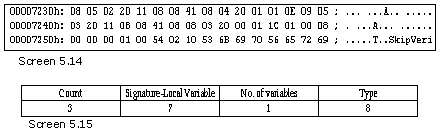As always, the first byte is the count byte. Based on the count, the bytes in the Blob area are copied into an array named blob1.

The second byte, which earlier contained the calling convention, now accommodates the value of 7, specifying Local Variables Signatures. If the value of the second byte is not 7, then an error is returned. The third byte is the count of the local variables. A 'for' loop iterates through all of them. As before, the function GetElementType does all the toiling. Since the value of the third byte is 8, the GetType function displays the output as int.

Now, we add a distinctive variable type to view the change in bytes and the output.

b.cs

public unsafe void abc()

{

int *i;

}

Output

Row 1

Count=4 Bytes 7 1 15 8 int *

In the file b.cs, there is a pointer to an int, which results in the type 15 in the signature. Since this is a Pointer type, we call the GetPointerValue method. This is akin to a class type, where the next byte is the actual data type. In this case, it is an int. We call the GetElementType method that reads the next byte and deciphers the type. A * symbol has been added to the string to denote a pointer.

b.cs

yyy a;

Output

Count=4 Bytes 7 1 17 12 yyy

The next is an object, which is of a value type yyy. This is similar to the class type, other than the type number 17. This calls a function named GetValueType, which works in a manner similar to the GetClassType function.

b.cs

yyy *a;

Output

Count=5 Bytes 7 1 15 17 12 yyy *

The GetElementType function works efficaciously. This is markedly evident from the output, where the variable is depicted as a pointer to a yyy type. The type 15 is a pointer type followed by the next number 17, which is a value type. This takes the token 12, which stands for the class yyy.

b.cs

xxx a = new xxx();

fixed ( int* i = &a.x )

{

}

Output

Count=7 Bytes 7 2 18 16 69 16 8 xxx , Pinned [ByRef] int

Two local variables named 'a' and 'i' are delineated in the above function. The variable 'a' is of type xxx. The first two bytes, i.e. 18 and 16 account for it. The number 69 is for the type named 'pinned', followed by 16 for the type named 'byref', and then 8, which is the final type of int.

'Pinning' is a medium by which the runtime is expressly instructed not to move a managed object around, since there is a pointer referencing it. By default, the runtime is permitted to move any object anywhere in memory.

b.cs

Object a;

Output

Count=3 Bytes 7 1 28 System.Object

The type 28 is reserved for System.Object. Similarly, the class of TypedReference has a number 22, IntPtr 24 and UintPtr 25.

b.cs

TypedReference a;

IntPtr b;

UIntPtr c;

Output

Count=5 Bytes 7 3 22 24 25 System.TypedReference  , System.IntPtr  , System.UintPtr

Field Signature

b.cpp

int (_stdcall *p)(int , char , float, double);

void main()

{

}

>cl /clr b.cpp

a.cs

public void DisplayFieldsTable()

{

int old = tableoffset;

bool b = tablepresent(4);

int offs = tableoffset;

tableoffset = old;

if ( b )

{

for ( int k = 1 ; k <= rows ; k++)

{

FieldAttributes flags = (FieldAttributes )BitConverter.ToInt16 (metadata, offs);

offs += 2;

offs += offsetstring;

offs += offsetstring;

Console.WriteLine("Row {0}",k );

Console.WriteLine("Name : {0}", GetString(name));

int count = blob[sig];

Console.WriteLine("Signature [{0}]:Count={1} ", sig , count);

string s = DisplayFieldsSignature (sig );

Console.WriteLine(s);

}

}

}

public string DisplayFieldsSignature(int index)

{

string  s = "Count=";

int cb; int uncompressedbyte;

int count;

cb = CorSigUncompressData(blob , index , out uncompressedbyte);

count = uncompressedbyte;

s = s + count.ToString() + " Bytes ";

for ( int l = 1; l <= count ; l++)

s = s + blob[index+l+cb-1].ToString() + " ";

byte [] blob1 = new byte[count];

Array.Copy(blob , index + 1 + cb -1  , blob1 , 0 , count);

index = 0;

cb = CorSigUncompressData(blob1 , index  , out uncompressedbyte);

if ( uncompressedbyte != 0x06)

{

s = "Error";

return s;

}

index = index + cb;

cb = CorSigUncompressData(blob1 , index  , out uncompressedbyte);

int cb1 ;

int bytes = uncompressedbyte;

s = s + " " + GetElementType(blob1 , bytes , index , out cb1);

return s;

}

public string GetElementType(byte [] b , int bytes , int index , out int cb1)

{

cb1 = 0;

string s = "";

if ( bytes <= 0x0e )

{

cb1 = 0;

s = GetType(bytes);

}

if ( bytes == 0x12 )

{

s = GetClassType(b , bytes , index , out cb1);

}

/*if ( bytes == 0x14 || bytes == 0x1d)

{

s = GetArrayType(b , bytes , index , out cb1);

}

*/

if ( bytes == 0x10)

{

s = GetReferenceType(b , bytes , index , out cb1);

}

if ( bytes == 0x0f)

{

s = GetPointerType(b , bytes , index , out cb1);

}

if ( bytes == 0x11 )

{

s = GetValueType(b , bytes , index , out cb1);

}

if ( bytes == 0x45 )

{

s = GetPinnedType(b , bytes , index , out cb1);

}

if ( bytes == 0x1c )

{

s = "System.Object ";

}

if ( bytes == 0x16 )

{

s = "System.TypedReference ";

}

if ( bytes == 0x18 )

{

s = "System.IntPtr ";

}

if ( bytes == 0x19 )

{

s = "System.UIntPtr ";

}

if ( bytes == 0x1b )

{

s = GetPointerToFunctionType (b , bytes , index , out cb1);

}

if ( bytes == 0x20 || bytes == 0x1f )

{

int uncompressedbyte;

int cb = CorSigUncompressData(b , index , out uncompressedbyte);

byte table = (byte)(uncompressedbyte & 0x03);

int ind = uncompressedbyte >> 2;

string s1= "";

if ( table == 1)

s1 = typerefnames[ind];

if ( table == 0)

s1 = typedefnames[ind];

index = index + cb;

int cb2 = CorSigUncompressData(b , index , out uncompressedbyte);

int cb3=0;

s = s1 + " " + GetElementType(b , uncompressedbyte , index, out cb3);

cb1 = cb + cb2 + cb3;

}

return s;

}

public string GetPointerToFunctionType(byte [] b , int bytes , int index , out int cb1)

{

string s = "";

int uncompressedbyte;

index = index + 1;

int cb = CorSigUncompressData(b , index , out uncompressedbyte);

index = index + cb;

s = GetCallingConvention(uncompressedbyte);

cb = CorSigUncompressData(b , index , out uncompressedbyte);

int noofparams = uncompressedbyte;

index = index + cb;

cb = CorSigUncompressData(b , index , out uncompressedbyte);

index = index + cb;

int cb2;

s = s + " " + GetElementType(b , uncompressedbyte , index , out cb2) ;

index = index + cb2;

s = s + "(";

for ( int l = 1 ; l <= noofparams ; l++)

{

cb = CorSigUncompressData(b , index , out uncompressedbyte);

index = index + cb;

s = s +  GetElementType(b , uncompressedbyte , index , out cb2) ;

if ( l != noofparams )

s = s + ",";

index = index + cb2;

}

s = s + ")";

cb1 = 0;

return s;

}

public string GetCallingConvention ( int uncompressedbyte )

{

int firstbyte = uncompressedbyte;

byte firstfourbits = (byte)(firstbyte & 0x0f);

string s = "\nCalling Convention ";

if ( firstfourbits == 0x00)

s = s + " DEFAULT ";

if ( firstfourbits == 0x01)

s = s + " C ";

if ( firstfourbits == 0x02)

s = s + " STDCALL ";

if ( firstfourbits == 0x03)

s = s + " THISCALL ";

if ( firstfourbits == 0x04)

s = s + " FASTCALL ";

if ( firstfourbits == 0x05)

s = s + " VARARG ";

if ( (firstbyte & 0x20) == 0x20)

s = s + " HASTHIS ";

if ( (firstbyte & 0x40) == 0x40)

s = s + " EXPLICIT ";

s = s + "\n";

return s;

}

public int ReadStringIndex(byte [] a , int o)

{

int z = 0;

if ( offsetstring == 2)

z = BitConverter.ToUInt16 (a , o );

if ( offsetstring == 4)

z = (int)BitConverter.ToUInt32 (a , o );

return z;

}

public string GetString(int starting)

{

int i = starting;

while (strings[i] != 0 )

{

i++;

}

System.Text.Encoding e = System.Text.Encoding.UTF8;

string  s = e.GetString(strings, starting , i - starting  );

return s;

}

Output

Row 1

Name : p

Signature :Count=13

Count=13 Bytes 6 27 2 4 32 17 8 8 32 25 4 12 13

Calling Convention  STDCALL

System.Runtime.CompilerServices.CallConvStdcall int(int,Microsoft.VisualC.NoSig

nSpecifiedModifier byte,float,double)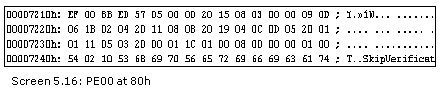The FillParamsArray has been employed in this program in order to fill up the typedefnames and typerefnames array. So, comment out the DisplayStandAloneSigTable and call the other two functions.

The FillParamsArray function, if it has been omitted in the program, it may be entered as follows:

//DisplayStandAloneSigTable();

FillParamsArray();

DisplayFieldsTable();

Furthermore, the DisplayElementType function has undergone transformation. Therefore, it has been reintroduced.  Moreover, the functions of ReadStringIndex and GetString are displayed, since they have been pressed into service in this program.

The file b.cpp is written in a language called managed C++, as a result of which, the file extension is cpp. The lone obligatory function is 'main', with a small letter 'm'. Besides the function main, there exists a field or global variable 'p', which is a pointer to a function. It takes four parameters, viz. an int, a char, a float and a double. Ultimately, it returns an int. The above program is compiled, using the managed C++ compiler cl with the option of /clr, in order to create a .Net file.

In the C# program, as was stated earlier, we have commented out the method DisplayStandAloneSigTable, and called the DisplayFieldsTable method instead. Using the method DisplayFieldsSignature, which is passed the index into the Blob stream, the name of the field and the signature have been displayed. If the signature following it happens to be a field signature, the first byte of the Blob heap must be 0x06. The GetElementType method merely returns this value.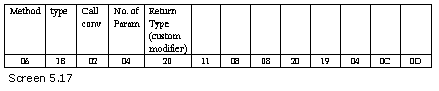All this while, we had to keep track of the position within the Blob array. This is not applicable here, since each field is represented by its own row in the Fields table. The actual code is contained in the GetElementType function since the type number for a pointer to a function is 27 or 0x1b.

We are reasonably more comfortable explaining the concept of 'pointers to functions' using managed C++, which is the language that we have earned our spurs on. The function GetPointerToFunctionType is called. The pointer to a function type is followed by the method signature. The first byte is the calling convention byte, since this is what a method signature typically commences with.

This byte has a value of 2, thereby indicating a Standard Calling convention. Our good old Windows programs used it ever so often. The GetCallingConvention method checks if the value of the calling convention is one of the following: C, Standard, ThisCall or FastCall.

The next byte is the number of parameters that the method takes, which is 4 in this case. The third is the return type of the method. This return type starts with the number 32, referred to by the documentation as a 'custom modifier'. A custom modifier starts out with either the optional modifier (like in our case), or with a fixed/required modifier 31. When the modifier is optional, the compiler holds the option of ignoring it. However, if the modifier is mandatory, it becomes obligatory on the part of the compiler to consider it.

The modifier has a token following it, which has already been expounded in considerable detail earlier. The GetElementType method is called again, with an additional check performed on the Required and Optional modifier. With the help of the token, the decoded class is displayed. The GetElementType method is invoked yet again, to decipher the next byte, which may not necessarily be a simple type.

A loop is implemented for a number of parameters. It hires the services of the GetElementType function to handle the custom modifier, since custom modifiers can also prevail upon the parameters. The first two parameters to the pointer are int and char. The first parameter has a custom modifier, while the second one does not.

Member Ref Table

b.cpp

int abc( int i , ...)

{

return 10;

}

void main()

{

abc(10);

abc(10,20);

abc(10,20,30);

}

a.cs

public void DisplayMemberRefTable()

{

int old = tableoffset;

bool b = tablepresent(10);

int offs = tableoffset;

tableoffset = old;

if ( b )

{

for ( int k = 1 ; k <= rows ; k++)

{

short clas = BitConverter.ToInt16 (metadata, offs);

offs += 2;

offs += offsetstring;

offs += offsetblob;

Console.WriteLine("Row {0}",k);

string s = GetString(name);

Console.WriteLine("Name:{0}" , s);

s = DisplayMethodSignature(sig, s);

Console.WriteLine(s);

}

}

}

public string DisplayMethodSignature(int index , string name)

{

string  s = "Count=";

int cb; int uncompressedbyte;

int count;

cb = CorSigUncompressData(blob , index , out uncompressedbyte);

count = uncompressedbyte;

s = s + count.ToString() + " Bytes ";

for ( int l = 1; l <= count ; l++)

s = s + blob[index+l+cb-1].ToString() + " ";

byte [] blob1 = new byte[count];

Array.Copy(blob , index + 1 + cb -1  , blob1 , 0 , count);

index = 0;

cb = CorSigUncompressData(blob1 , index  , out uncompressedbyte);

s = s + GetCallingConvention(uncompressedbyte);

int paramcount;

index = index + cb;

cb = CorSigUncompressData(blob1 , index  , out uncompressedbyte);

paramcount = uncompressedbyte;

s = s + "Number of Parameters " + paramcount.ToString() + "\n";

index = index + cb;

cb = CorSigUncompressData(blob1 , index  , out uncompressedbyte);

string s1;

s1 = GetReturnType( blob1 , uncompressedbyte , index , out cb);

s = s + "Return Type:" + s1 + "\n";

index = index + cb + 1;

s = s + "Signature " + name + "(";

for ( int l = 1 ; l <= paramcount ; l++)

{

cb = CorSigUncompressData(blob1 , index  , out uncompressedbyte);

int cb1 ;

int bytes = uncompressedbyte;

if ( bytes == 65)

{

s = s + "...";

index = index + 1;

l--;

continue;

}

s1 = GetElementType(blob1 , bytes , index , out cb1);

index = index + cb + cb1;

s = s + " " + s1;

if ( l != paramcount )

s = s + ",";

}

s = s + ")\n";

return s;

}

public string GetReturnType(byte [] b , int uncompressedbyte , int index , out int cb)

{

string s = "";

if (uncompressedbyte == 32)

index = index + 1;

s = GetElementType(b, uncompressedbyte , index , out cb);

return s;

}

Output

Row 1

Name:.ctor

Count=5 Bytes 32 1 1 17 5

Calling Convention  DEFAULT  HASTHIS

Number of Parameters 1

Return Type:void

Signature .ctor( System.Security.Permissions.SecurityAction)

Row 2

Name:abc

Count=8 Bytes 5 2 32 17 8 8 65 8

Calling Convention  VARARG

Number of Parameters 2

Return Type:System.Runtime.CompilerServices.CallConvCdecl int

Signature abc( int,... int)

Row 3

Name:.ctor

Count=4 Bytes 32 1 1 14

Calling Convention  DEFAULT  HASTHIS

Number of Parameters 1

Return Type:void

Signature .ctor( string)

Row 4

Name:abc

Count=9 Bytes 5 3 32 17 8 8 65 8 8

Calling Convention  VARARG

Number of Parameters 3

Return Type:System.Runtime.CompilerServices.CallConvCdecl int

Signature abc( int,... int, int)

Row 5

Name:abc

Count=6 Bytes 5 1 32 17 8 8

Calling Convention  VARARG

Number of Parameters 1

Return Type:System.Runtime.CompilerServices.CallConvCdecl int

Signature abc( int)

Row 6

Name:.ctor

Count=3 Bytes 32 0 1

Calling Convention  DEFAULT  HASTHIS

Number of Parameters 0

Return Type:void

Signature .ctor()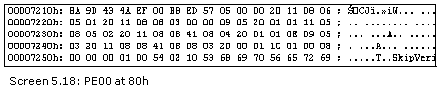The MemberRef table gets populated with the details of each and every function that is called in the program. In the managed C++ program, the three dots in the code are used to indicate a variable number of arguments. However, it is essential to specify the first parameter for the function, which may be called subsequently, with any number of parameters.

In the above program named b.cpp, the function abc is called from main, with one, two and three parameters. This adds three records to the MemberRef table.

The function DisplayMemberRefTable is called explicitly after calling the FillParamsArray function. Using this function, all the methods are displayed. However, for explanation purposes, we will only consider the method abc, and not the constructors. The method DisplayMethodSignature is used to decode the signature in the Blob heap. Therefore, it is provided with the index and the name.

We have deliberately evaded the coded index and have used the integer types for the parameters. Therefore, the value of 8 is flashed across. The number 32 is the optional modifier that is followed by the token, and hence, this too would be ignored.

The first call of the abc method has two integers, out of which, one is optional and the other is mandatory. It is located in the second row of the member ref table. The last three bytes of the signature are also displayed.

We had made the presumption that the signature would end with two 8's. However, the number 65 is visible in the middle. This byte is called the 'sentinel'. It signifies that all the subsequent parameters are optional. Therefore, we display three dots when the sentinel byte occurs.

Row 4 shows the sentinel byte 65 followed by two 8's. This is because the abc function is called with two optional ints. The last row has a single int. Thus, there are no optional parameters, as a result of which, there is no sentinel byte.

While coding, we discovered that code could be introduced for the sentinel byte in our GetElementType method. This is because the loop relies upon the paramcount variable.

This is not all. Nurturing the sentinel byte in the GetElementType function leads to an increase in the param count too.

The sentinel handling code that we have introduced is absolutely straightforward. We decrease the loop variable l by 1. Then, we add three dots to the string s. Next, we increase the index variable by 1, since the next byte is to be read. Finally, using the 'continue' statement, we revert to the start of the 'for' loop.

It would be a much healthier option to alter the index of the 'for' loop, from paramcount to the number of bytes in the signature.